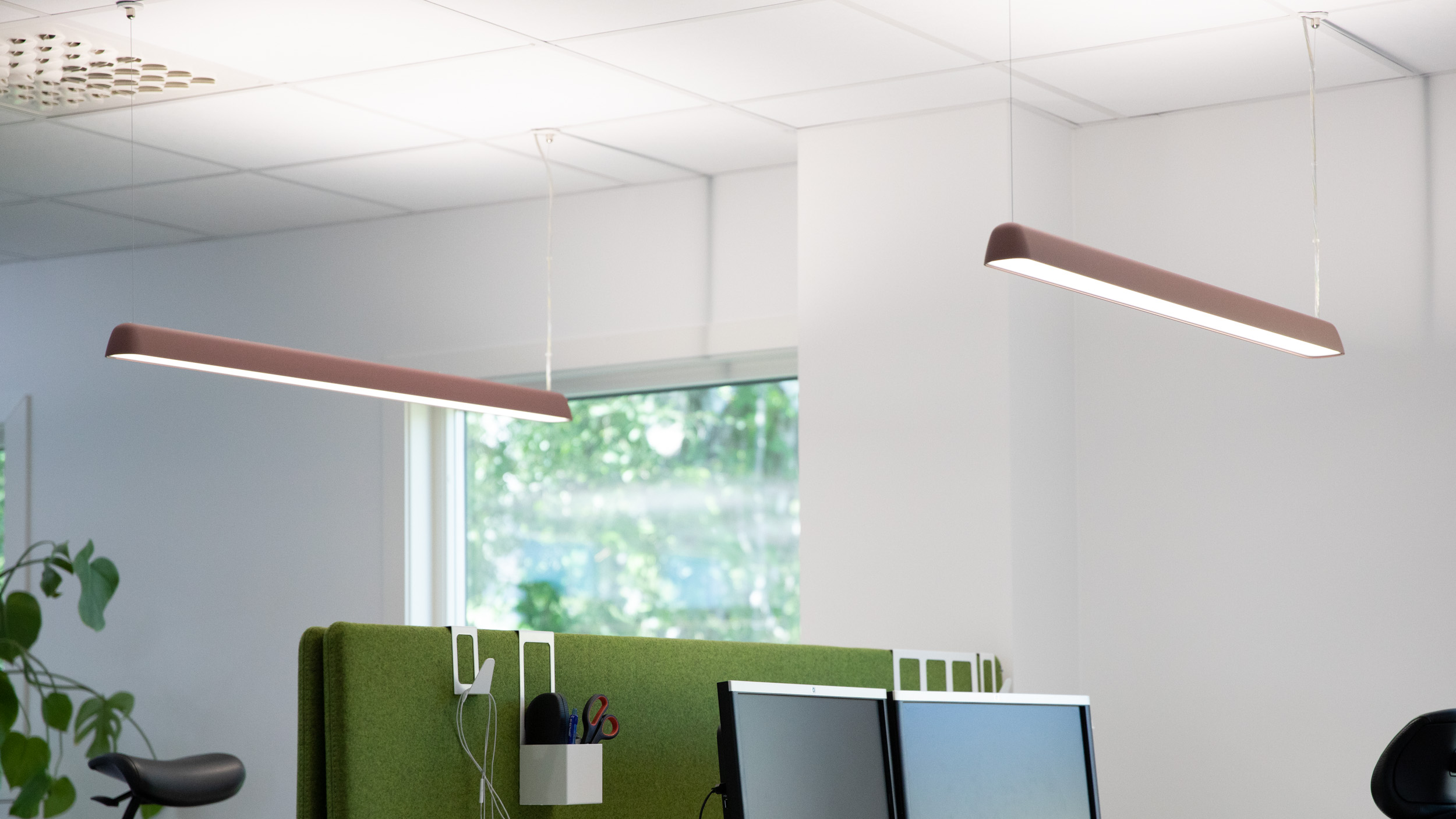Calculation areas

# Number of calculation points

A grid of calculation points shall be created to calculate and check the average illuminance and uniformity of illuminance in the working area, the immediate surrounding area and the peripheral surrounding area.The minimum number of calculation points to be used can be calculated using the formula shown below.

Note that the distances and locations of the calculation points should not coincide with the distances between the luminaires in the room. A grid that approaches the shape of a box or square is to be preferred, and the relationship between the dimensions of the length and width of the grid should be kept within the range 0.5–2.

The formula for calculating the maximum distance between calculation points in the grid is as follows:

• P = 0.2×5 log d
• Where p <10
• d – is the longer dimension for the surface
• p – is the maximum distance between the calculation points

A strip of maximum 0.5 meters from each external wall can be excluded from the calculation except when a working area falls within this zone. The size of the grid for walls and ceilings is calculated in a similar way and, here as well, a band of 0.5 m can be excluded from the respective outer edge.

Below is a list of examples of maximum distances between calculation points according to the above formula, based on the size and length of the working area.

 Length of surface Maximum distance between calculation points 0.40 0.15 m or at least 3 points 0.60 m 0.20 m or at least 3 points 1.00 m 0.20 m or at least 5 points 2.00 m 0.30 m or at least 6 points 5.00 m 0.60 m or at least 8 points 10.00 m 1.00 m or at least 10 points 25.00 m 2.00 m or at least 12 points 50.00 m 3.00 m or at least 17 points 100.00 m 5.00 m or at least 20 points

### Examples of the number of calculation points for checking illuminance and uniformity in classrooms and office workplaces

Working area in a standard classroom, without workplaces at walls.

Supplement with a separate working area within the wall zone. The working area and immediate surrounding area is checked.

Defined working area of 0.6 m in a cellular office with immediate surrounding area as a strip around the working area with a width of at least 0.5 m.As well as an outer surrounding area such as a strip around the immediate surrounding area of at least 3 m, but where the peripheral surrounding area touches the wall, the peripheral surrounding area is limited by a zone of 15% of the shortest dimension of the room, but a maximum of 0.5 m from the walls of the room. The requirement for cylindrical illuminance is calculated here for the area corresponding to the size of the working area and immediate surrounding area.